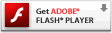a â€śheld to maturity investmentsâ€ť mainly comprise bonds and other money market securities intended to be held to maturity. They are initially recognized at fair value and are subsequently measured at amortized cost at each balancesheet date. If there is an objective indication of impairment, an impairment loss is recognized at the balance-sheet date. The impairment loss corresponds to the difference between the carrying amount and the recoverable amount (i.e. the Present value of the expected cash flows discounted using the original effective interest rate) and is recognized in profit or loss. This loss may be reversed if the recoverable amount increases in a subsequent period.

For these two categories, initial fair value is equivalent to acquisition cost, because no material transaction costs are incurred;

a â€śavailable-for-sale financial assetsâ€ť mainly comprise investments in non-consolidated companies, equities, mutual fund units and money market securities. These assets are measured at fair value, with changes in fair value recognized in equity. The fair value of listed securities corresponds to market price (level 1 valuation technique) and the fair value of unlisted equities and mutual funds corresponds to their net asset value (level 1 valuation technique). For unlisted securities, fair value is estimated based on the most appropriate criteria applicable to each individual investment (using level 3 valuation techniques that are not based on observable data). Securities that are not traded on an active market, for which fair value cannot be reliably estimated, are carried in the balance sheet at historical cost plus any transaction expenses. When there is objective evidence of a significant or prolonged decline in value, the cumulative unrealized loss recorded in equity is reclassified to the income statement and can not be reversed.

N.2. Derivative financial Instruments

Derivative financial instruments such as interest rate and currency swaps, caps and forward purchases of foreign currencies, are used solely to hedge exposures to changes in interest rates and exchange rates.

Financial statements

CONSOLIDATED FINANCIAL STATEMENTS

5

They are measured at fair value. Changes in fair value are recognized in profit, except for instruments qualified as cash flow hedges (hedges of variable rate debt) for which changes in fair value are recognized in equity.

The fair value of interest rate derivatives is equal to the present value of the instrumentâ€™s future cash flows, discounted at the interest rate for zero-coupon bonds.

The fair value of currency derivatives is determined based on the forward exchange rate at the period-end.

N.3. Financial liabilities hedged by derivative instruments

Financial liabilities hedged by derivative instruments qualify for hedge accounting. The derivative instruments are classified as either fair value hedges or cash flow hedges.

Financial liabilities hedged by fair value hedges are measured at fair value, taking into account the effect of changes in interest rates. Changes in fair value are recognized in profit and are offset by changes in the fair value of the hedging instrument.

Financial liabilities hedged by cash flow hedges are measured at amortized cost. Changes in the fair value of the hedging instrument are accumulated in equity and are reclassified into profit in the same period or periods during which the financial liability affects profit.

N.4. Bank borrowings

Interest-bearing drawdowns on lines of credit and bank overdrafts are recognized for the amounts received, net of direct issue costs.

N.5. Convertible bonds

Convertible bonds are qualified as hybrid instruments comprising a host contract, recognized in debt, and an embedded derivative, recognized in equity.

The carrying amount of the host contract or debt component is equal to the present value of future principal and interest payments, discounted at the rate that would be applicable to ordinary bonds issued at the same time as the convertible bonds, less the value of the conversion option calculated at the date of issue.